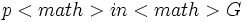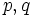# Proving intersection-closedness

This is a survey article related to:subgroup metaproperty satisfaction
View other survey articles about subgroup metaproperty satisfaction

A subgroup property$p$ is termed an intersection-closed subgroup property if an arbitrary (nonempty) intersection of subgroups having property$p$ also has property$p$.$p$ is termed a strongly intersection-closed subgroup property if it is intersection-closed and is also an identity-true subgroup property -- it is satisfied by every group as a subgroup of itself.$p$ is termed a finite-intersection-closed subgroup property if the intersection of finitely many subgroups satisfying the property$p$ also has the property$p$.$p$ is a strongly finite-intersection-closed subgroup property if it is finite-intersection-closed and identity-true.

This article discusses techniques to prove that a given subgroup property is intersection-closed.

Also refer:

## Invariance properties

Further information: Invariance implies strongly intersection-closed

Suppose$a$ is a property of functions from a group to itself. The invariance property corresponding to$a$ is defined as the following property$p$:$H$ has property$p$ in$G$ if every function from$G$ to itself satisfying property$a$ sends$H$ to within itself.

Invariance properties are strongly intersection-closed. In other words, they are closed under arbitrary intersections, and every group satisfies the property as a subgroup of itself.

Here are some examples:

## Left-hereditary subgroup properties

Further information: Left-hereditary implies intersection-closed

A subgroup property$p$ is termed a left-hereditary subgroup property if, whenever$H$ is a subgroup of a group$G$ satisfying property$p$ in$G$, any subgroup$K$ of$H$ also satisfies property$p$ in$G$.

Left-hereditary subgroup properties are intersection-closed for obvious reasons. However, a left-hereditary subgroup property is not identity-true unless it is the tautology. Hence, it is not a strongly intersection-closed subgroup property.

Some examples are:

## Galois correspondences

Some subgroup properties arise as a result of Galois correspondences. We cal such a property a Galois correspondence-closed subgroup property.

We start with a rule which, for every group, gives a binary relation between the group and another set constructed canonically from the group. The rule must be isomorphism-invariant, in the sense that any isomorphism of groups respects the binary relation.

The subgroup property we now get is the property of being a subgroup, which is also a closed subset of the group under the Galois correspondence induced by the binary relation.

Any Galois correspondence-closed subgroup property is strongly intersection-closed. Some examples are:

## Property of a normal subgroup based on the isomorphism class of its quotient group

Suppose$a$ is a group property and$p$ is the property of being a normal subgroup of a group for which the quotient group has property$a$. Then:

• If$a$ is closed under taking finite subdirect products, then$p$ is finite-intersection-closed. In particular, for instance, if$a$ is a [quasivarietal group property]],$p$ is strongly finite-intersection-closed.
• If$a$ is closed under arbitrary subdirect products, then$p$ is intersection-closed. In particular, for instance, if$a$ is a varietal group property,$p$ is a strongly intersection-closed subgroup property.

## Effect of logical operators

### Conjunction

Further information: Intersection-closedness is conjunction-closed

If$p$ and$q$ are both intersection-closed subgroup properties, so is the conjunction (AND) of$p$ and$q$. More generally, the conjunction of an arbitrary collection of intersection-closed subgroup properties is intersection-closed.

Note that in some cases, one of the properties in the conjunction is a group property interpreted as a subgroup property. In this case, it suffices to show that the group property is a subgroup-closed group property and the subgroup property is intersection-closed.

Analogous observations apply to strongly intersection-closed, finite-intersection-closed, and strongly finite-intersection-closed subgroup properties.

Some examples of conjunctions of intersection-closed properties that continue to be intersection-closed:

## Effect of subgroup property modifiers

In this section, we discuss various subgroup property modifiers and their impact on the metaproperty of being intersection-closed and finite-intersection-closed.

### Intersection-transiter

By definition, the intersection-transiter of any subgroup property is a strongly finite-intersection-closed subgroup property. In other words, it is satisfied by every group as a subgroup of itself and is closed under all finite intersections.

### Intersection-closure operator

Here are some facts:

### Intersection operator

The intersection operator takes as input two subgroup properties$p$ and$q$, and outputs the property$p \cap q$, defined as follows. A subgroup$H$ of a group$G$ satisfies property$p \cap q$ in$G$ if there are subgroups <math<K,L[/itex] of$G$ such that$K$ satisfies$p[itex] in [itex]G$,$L$ satisfies$q$ in$G$, and$H = K \cap L$.

Here are some facts:

• Intersection operator preserves finite-intersection-closedness: If$p,q$ are both finite-intersection-closed, so is$p \cap q$.
• Intersection operator preserves intersection-closedness: If$p,q$ are both intersection-closed, so is$p \cap q$.

Analogous results hold for strongly finite-intersection-closed and strongly intersection-closed.

### Left residual, left transiter

Let$p$ and$q$ be subgroup properties. The left residual of$p$ by$q$ is the following subgroup property$r$: a subgroup$H$ of a group$G$ satisfies property$r$ in$G$ if, for any group$K$ containing$G$ as a subgroup with property$p$,$K$ contains$H$ with property$p$.

It turns out that if$p$ is intersection-closed, so is the left residual of$p$ by$q$. Analogous observations hold for strongly intersection-closed, finite-intersection-closed.

The left transiter of a subgroup property is its left residual by itself. The above result shows that the left transiter of any intersection-closed subgroup property is intersection-closed.

Here are some examples:

### Transfer condition operator

Further information: Transfer condition operator preserves intersection-closedness

The transfer condition operator$T$ is defined as follows. For a subgroup property$p$,$T(p)$ is defined as follows: a subgroup$H$ of a group$G$ satisfies property$T(p)$ if, for any subgroup$K$ of$G$,$H \cap K$ satisfies property$T(p)$ in$K$.